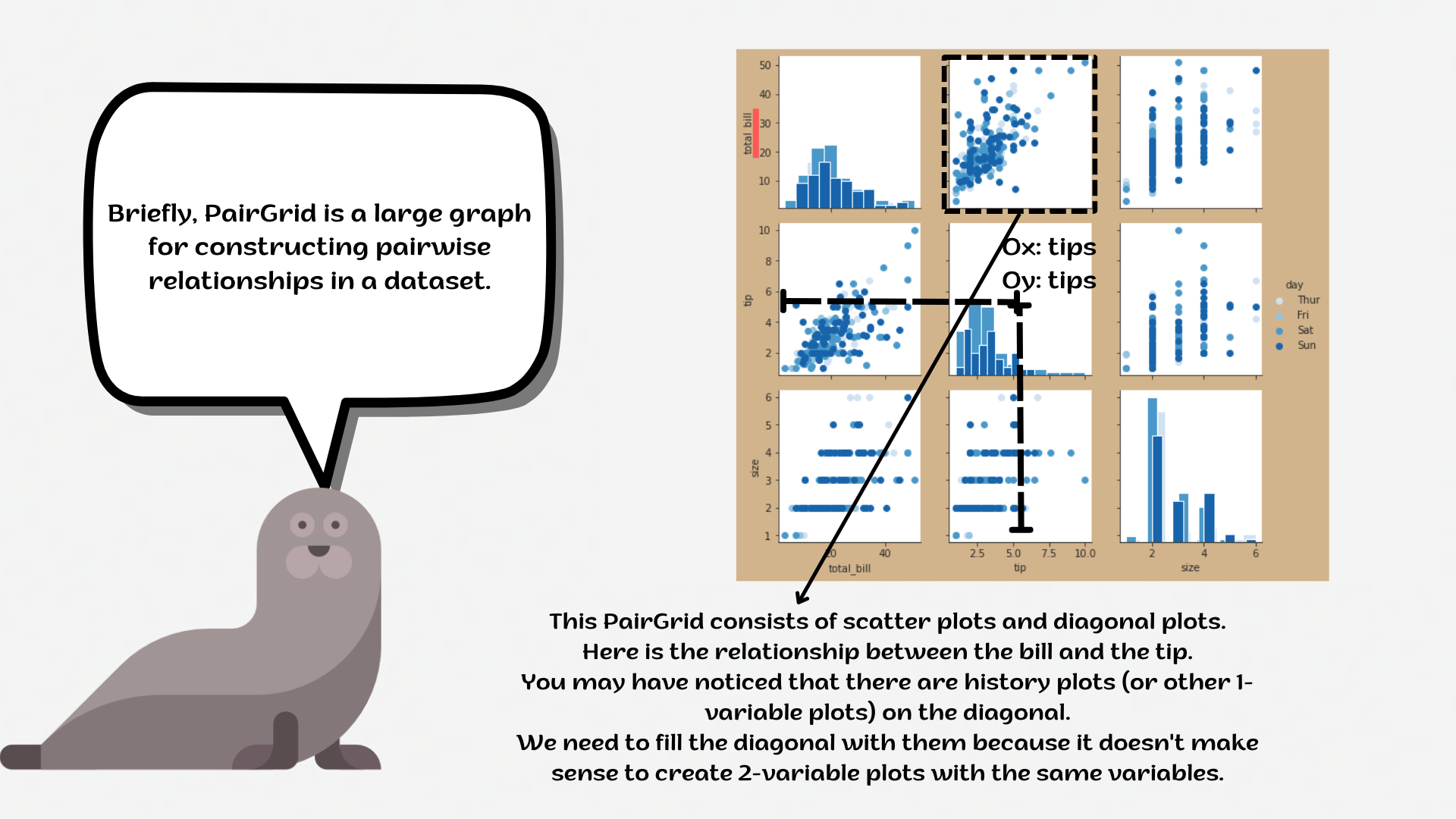Course Content

# Deep Dive into the seaborn Visualization

1. Light Start

2. Distributions of Data

3. Categorical Plot Types

4. Matrix Plots

5. Multi-Plot Grids

6. Regression Models

Deep Dive into the seaborn Visualization

##PairGrid`PairGrid` is a subplot grid for plotting pairwise relationships in a dataset.

This object maps each variable in a dataset onto a column and row in a grid of multiple axes. Different axes-level plotting functions can be used to draw bivariate plots in the upper and lower triangles, and then the marginal distribution of each variable can be shown on the diagonal.1. Set the `'ticks'` style with the `'lightpink'` `figure.facecolor`.
2. Create a `PairGrid` variable using `g`:
• Set the data for the `g`;
• Set the `hue` parameter equals the `'species'`;
• Set the `'rocket_r'` palette.

Set diagonale plots using the `.map_diag()` function:

• Create a `histplot` using the `seaborn`;
• Add the `kde` parameter.

Set non-diagonale plots using the `.map_offdiag()` function:

• Create a `scatterplot` using the `seaborn`;
• Set the `linewidth` parameter equals `0.9`;
• Set the `'purple'` `edgecolor` parameter.

Everything was clear?

Section 5. Chapter 2